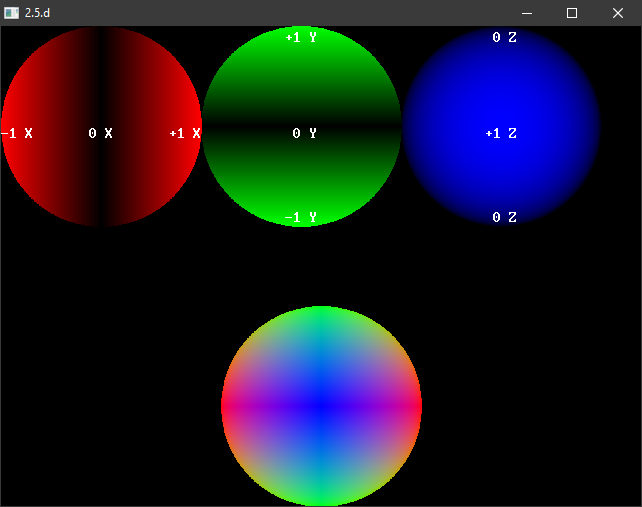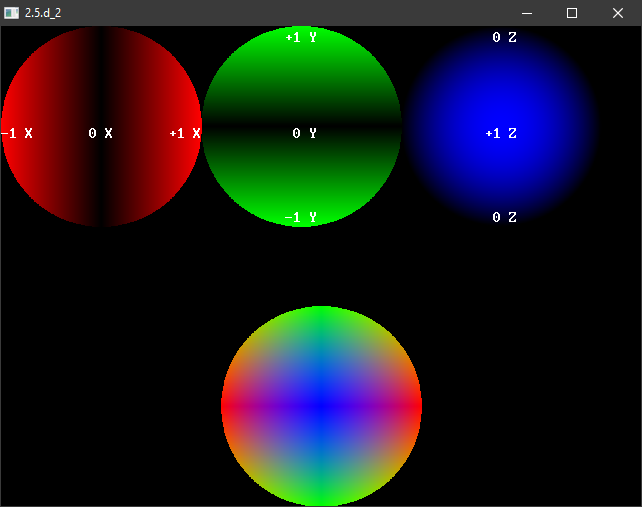## Search found 7736 matches

Sep 27, 2020 13:36
Forum: General
Topic: Project of a multiplatform version of FreeBasic Miner
Replies: 35
Views: 950

### Re: Project of a multiplatform version of FreeBasic Miner

On most Linux 32-bit distros:
you need libfbsound-32.so and libplug-alsa-32.so

On most Linux 64-bit distros:
you need libfbsound-64.so and libplug-alsa-64.so

Joshy
Sep 27, 2020 6:22
Forum: General
Topic: Does the behavior of compile switch -w all changed ?
Replies: 7
Views: 145

### Re: Does the behavior of compile switch -w all changed ?

So it crashed and gives no warning of running out of stack space.

How should a beginner find this mistake ?

looks for me as an "new" bug !

Joshy
Sep 27, 2020 6:19
Forum: General
Topic: Project of a multiplatform version of FreeBasic Miner
Replies: 35
Views: 950

### Re: Project of a multiplatform version of FreeBasic Miner

Roland Chastain wrote:@D.J.Peters
I don't know if all FBSound files are really needed. The program uses only WAV files. If you have time to take a look...
What do you mean with "all FBSound files" FBsound need no audio file at all the files included in FBsound are for the tests and demos !

Joshy
Sep 27, 2020 4:30
Forum: General
Topic: Does the behavior of compile switch -w all changed ?
Replies: 7
Views: 145

### Re: Does the behavior of compile switch -w all changed ?

@coderJeff does it crash on your box or not ?

Joshy
Sep 27, 2020 2:56
Forum: General
Topic: Does the behavior of compile switch -w all changed ?
Replies: 7
Views: 145

### Re: Does the behavior of compile switch -w all changed ?

I don't get any warning here ! vect3 is 16 bytes not 12 ! FreeBASIC Compiler - Version 1.08.0 (2020-08-15), built for win32 (32bit) Copyright (C) 2004-2019 The FreeBASIC development team. standalone Joshy type vec3 as single x,y,z as single pad ' 16 bytes end type const TSIZE = 256 const TMASK = TSI...
Sep 27, 2020 2:28
Forum: General
Topic: Does the behavior of compile switch -w all changed ?
Replies: 7
Views: 145

### Does the behavior of compile switch -w all changed ?

I used a 2D grid to create a 3d terrain something like: const GRID_SIZE = 64 const GRID_MASK = GRID_SIZE -1 dim as vector3 grid(GRID_MASK, GRID_MASK) Than I changed GRID_SIZE = 256 and the program crashed. To fix it i use shared: dim shared as vector3 grid(GRID_MASK, GRID_MASK) If I compile with -w ...
Sep 27, 2020 2:19
Forum: Community Discussion
Topic: screeninfo integers
Replies: 4
Views: 165

### Re: screeninfo integers

Using the native integer is a feature not a limitation !

Joshy
Sep 25, 2020 23:06
Forum: Community Discussion
Topic: [solved] if x2+y2 <= 1 then z2=1-(x2+y2) : z=sqr(z2) ?
Replies: 10
Views: 285

### Re: if x2+y2 <= 1 then z2=1-(x2+y2) : z=sqr(z2) ?

Thank you all for your hints. z = 1 - (d2*d2)/srq(2) is not perfect but good as replacement for z = sqr(z2) and positive also (I removed abs() fom color term) RGB(abs(x)*255, abs(y)*255, z*255) I was really thinking the information of z are aviable in the known numbers and I'm only blind to see it B...
Sep 25, 2020 3:21
Forum: Community Discussion
Topic: Reinventing the wheel
Replies: 17
Views: 581

### Re: Reinventing the wheel

I hope this 58 seconds give you an idea what I think about "do what you want to do !"

youtube: 58 seconds from Stan Lee

Joshy
Sep 25, 2020 1:20
Forum: Community Discussion
Topic: [solved] if x2+y2 <= 1 then z2=1-(x2+y2) : z=sqr(z2) ?
Replies: 10
Views: 285

### Re: if x2+y2 <= 1 then z2=1-(x2+y2) : z=sqr(z2) ?

distance squared = x*x + y*y z = (1*1) - (distance_squared *distance_squared ) / 1.414213562 = z = 1 - (distance_squared *distance_squared ) / 1.414213562 a2 + b2 = c2 1*1 + 1*1 = 2 1.414213562 = sqr(2) Is 99% accurat let me know if you find a better solution and thank you for your tips. Joshy EDIT:...
Sep 25, 2020 1:00
Forum: Community Discussion
Topic: [solved] if x2+y2 <= 1 then z2=1-(x2+y2) : z=sqr(z2) ?
Replies: 10
Views: 285

### Re: if x2+y2 <= 1 then z2=1-(x2+y2) : z=sqr(z2) ?

screenres 640,480,32 dim as integer w,h screeninfo w,h width w/8,h/16 dim as single r = 100, r2=r*r dim as single cx = w/2.0, cy = h/2.0 dim as single sx=2.0/w dim as single sy=2.0/h for y as single = -1 to 1 step sy dim as single y2=y*y for x as single = -1 to 1 step sx dim as single x2 = x*x dim ...
Sep 24, 2020 23:43
Forum: Community Discussion
Topic: [solved] if x2+y2 <= 1 then z2=1-(x2+y2) : z=sqr(z2) ?
Replies: 10
Views: 285

### Re: if x2+y2 <= 1 then z2=1-(x2+y2) : z=sqr(z2) ?

badidea wrote:But I see no way to get z without using sqr().
Stand on my shoulder and you will see much more as before :-)

I don't give up at this early stage :-)

Joshy
Sep 24, 2020 23:35
Forum: Tips and Tricks
Topic: Simple FBImage / Sprite scaling in Basic
Replies: 5
Views: 1745

### Re: Simple FBImage / Sprite scaling in Basic

Fixed a small bug (integer vs ulong color) and added fps counter.

Joshy
Sep 24, 2020 22:38
Forum: Community Discussion
Topic: [solved] if x2+y2 <= 1 then z2=1-(x2+y2) : z=sqr(z2) ?
Replies: 10
Views: 285

### Re: if x2+y2 <= 1 then z2=1-(x2+y2) : z=sqr(z2) ?

sqr()fake compare the blue z values not bad but not the right!Sep 24, 2020 22:30
Forum: Community Discussion
Topic: [solved] if x2+y2 <= 1 then z2=1-(x2+y2) : z=sqr(z2) ?
Replies: 10
Views: 285

### [solved] if x2+y2 <= 1 then z2=1-(x2+y2) : z=sqr(z2) ?

if x2+y2 <= 1 then z2 = 1-(x2+y2) : z = sqr(z2) How to remove the sqr() to get z ? I know there must be a simple 2D solution we know: radius and radius squared both 1 (Unit Circle) the range of x: = -1 -> 0 -> +1 the range of y: = -1 -> 0 -> +1 x squared x2 = x*x y squared y2 = y*y z squared z2 = 1 ...